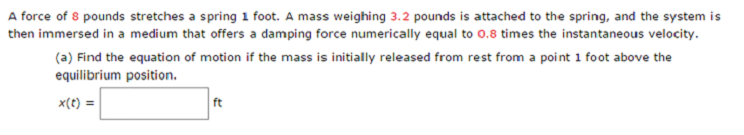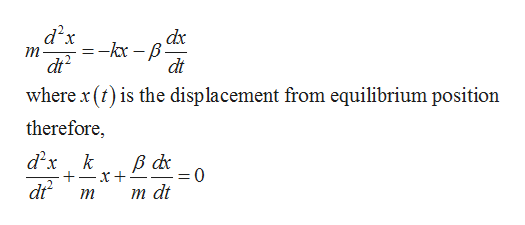# A force of 8 pounds stretches a spring 1 foot. A mass weighing 3.2 pounds is attached to the spring, and the system isthen immersed in a medium that offers a damping force numerically equal to 0.8 times the instantaneous velocity.(a) Find the equation of motion if the mass is initially released from rest from a point 1 foot above theequilibrium position.x(t)ft

Question
109 viewshelp_outlineImage TranscriptioncloseA force of 8 pounds stretches a spring 1 foot. A mass weighing 3.2 pounds is attached to the spring, and the system is then immersed in a medium that offers a damping force numerically equal to 0.8 times the instantaneous velocity. (a) Find the equation of motion if the mass is initially released from rest from a point 1 foot above the equilibrium position. x(t) ft fullscreen
check_circle

Step 1

Let m be the mass attached and k is the string constant and let β be the positive damping constant. The newton second law for the system is:help_outlineImage Transcriptionclosed x ,dx т dt where x () is the displacement from equilibrium position dt therefore, d x k 0 dr т at т fullscreen
Step 2

Now, find the equation of motion. First, convert the weight into unit of masses:

Step 3

Now, find spring c...

### Want to see the full answer?

See Solution

#### Want to see this answer and more?

Solutions are written by subject experts who are available 24/7. Questions are typically answered within 1 hour.*

See Solution
*Response times may vary by subject and question.
Tagged in

### Math